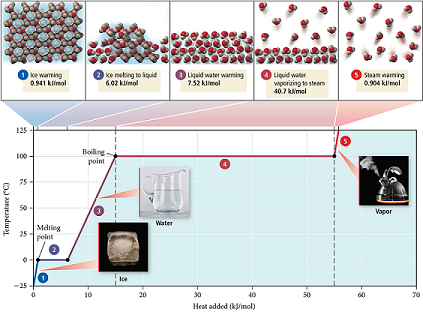# Problem: Refer to the figure below to answer each question.In moving from left to right along the line segment labeled 2 on the graph, heat is absorbed, but the temperature remains constant. Where does the heat go?

🤓 Based on our data, we think this question is relevant for Professor Tyson's class at UMASS.

###### FREE Expert Solution

We have to determine why the temperature remains constant at segment marked 2 in the given diagram.

We can see that the segment marked 2 is at the melting point of water.###### Problem Details

Refer to the figure below to answer each question.In moving from left to right along the line segment labeled 2 on the graph, heat is absorbed, but the temperature remains constant. Where does the heat go?